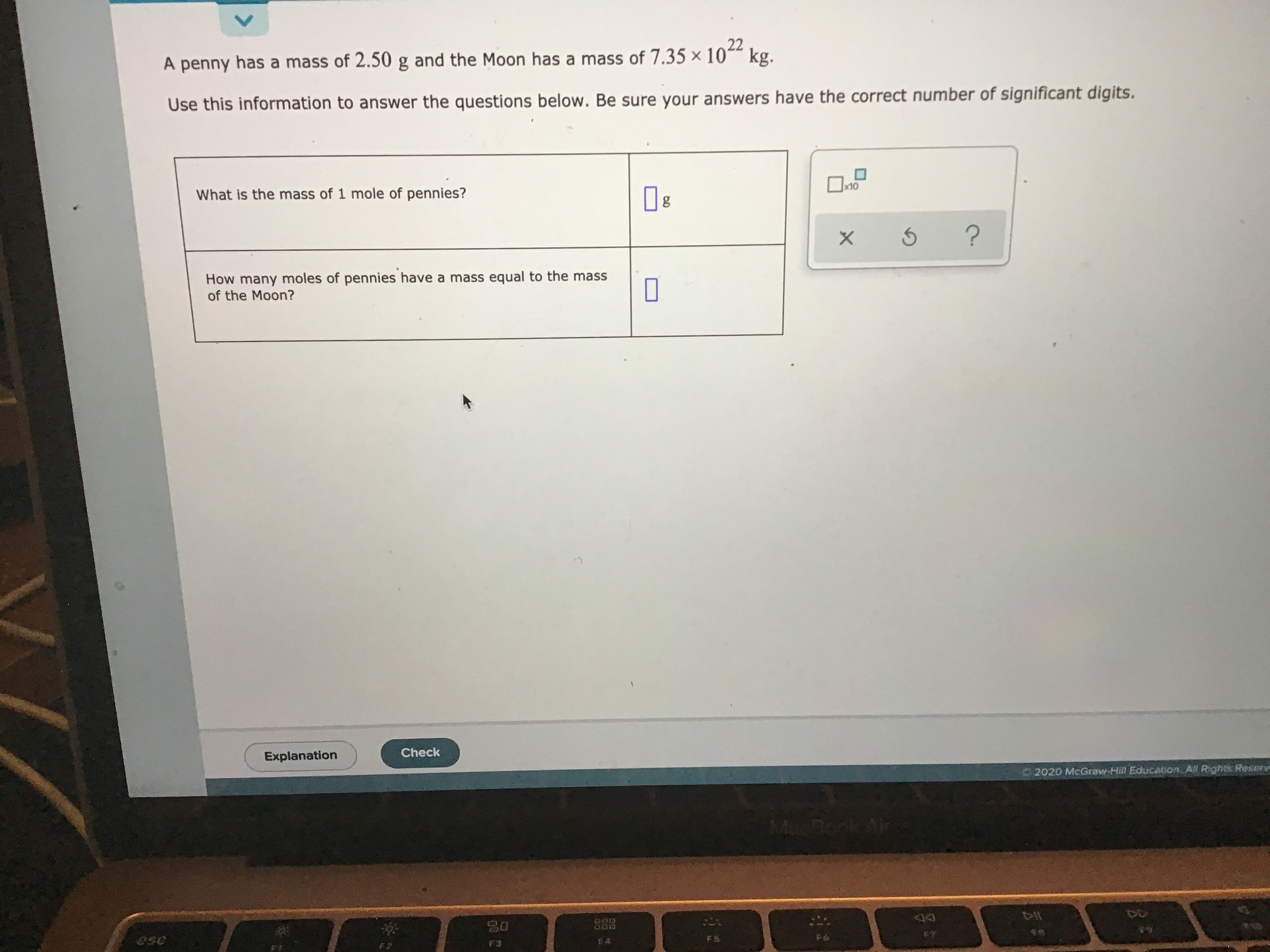# A penny has a mass of 2.50g and the Moon has a mass of 7.35×10^22 kg. Use this information to answer the question below. Be sure your answers have the correct number of significant digits. What is the mass of 1 mole of pennies? How many moles of pennies have a ass equal to the mass of the Moon?